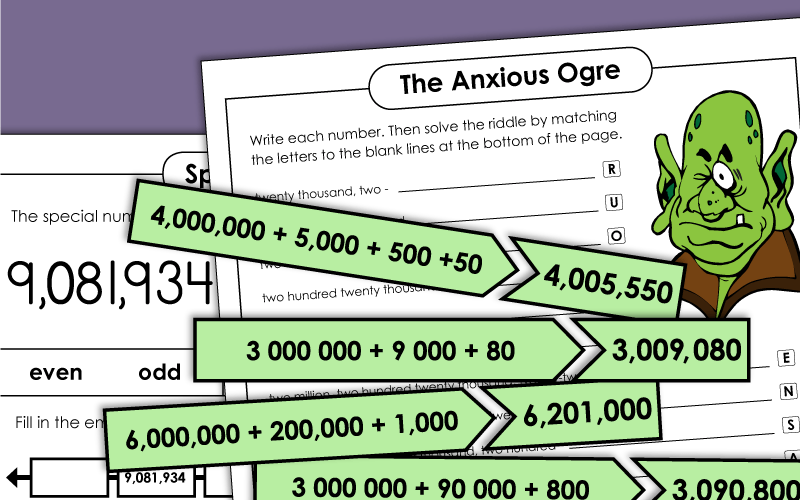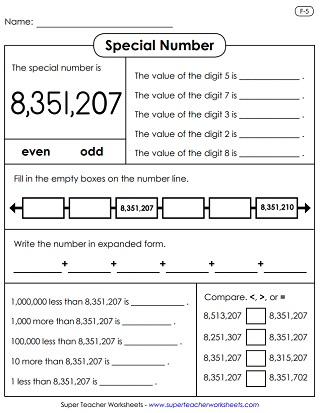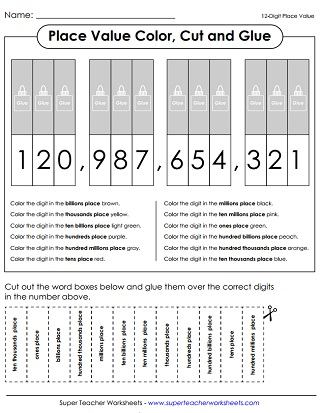# Place Value: 7-Digits (Millions)

This page has place value worksheets for large numbers with 7 digits (millions). Use these printables to teach ordering numbers, expanded notation, and adding commas to large numbers.## Place Value

Read each 7-digit number and write the value of the underlined digit.
Make large numbers even larger by writing them in expanded form; up to millions place.
Cut the place values and glue them above the digits, then color the numbers according to the directions; up to millions.
Cut the rectangles and match the equivalent numbers. Then tell whether each is written in expanded form, word name, or standard form.
Match each number with its expanded form to complete each puzzle. Numbers go up to the millions place.
What do you call an anxious ogre? To complete the math riddle, students must write the numbers in standard form. Then match the numbers with the letters.
Students are given digits.  Students arrange the digits to make the numbers given by the teacher.
Read the numbers, then tell the place and value of the underlined digit; Up to 10 millions.
Look at the numbers and add commas in the correct places; Numbers up to hundred millions
Cut the rectangles and match the equivalent numbers. Then tell whether each is written in expanded form, word name, or standard form.

## P.V. Chart

Practice creating and reading huge 7-digit numbers with this place value table.

## Special Numbers

On this worksheet, students answer questions related to the special number. First, they state the value of the digits 0, 2, 9, 7, and 4. Then tell if the number is odd or even. Complete the number line. Write the number in expanded form. Subtract a thousand from this number. Then compare the number to other numbers.
Use the symbols <, >, and = to compare this number to other numbers. Find the values of the digits 3, 1, 2, 9, and 7. Tell what numbers come before and after by completing the number line. Write in expanded form.
This worksheet has a set of place value questions to go along with the number nine million, eighty-one thousand, nine hundred thirty-four.
Like the other special number worksheets, this one covers expanded form, a number line, even/odd, comparing, adding, subtracting, and questions about digit values.
Answer the place value questions related to the number eight million, three hundred fifty-one thousand, two hundred seven.

## Ordering Numbers

Each box has a set of five numbers. Students must rewrite them in order, starting with the number that has the smallest value.

Say the names of the 7-digit numbers.  Includes a homework sheet and a test.
Decimal and Money Place Value

Learn about decimal numbers, with place values for tenths, hundredths, and thousandths.

Rounding Numbers

Round numbers to the nearest tens, hundreds, and thousands place.

## Worksheet ImagesMy Account
Site Information